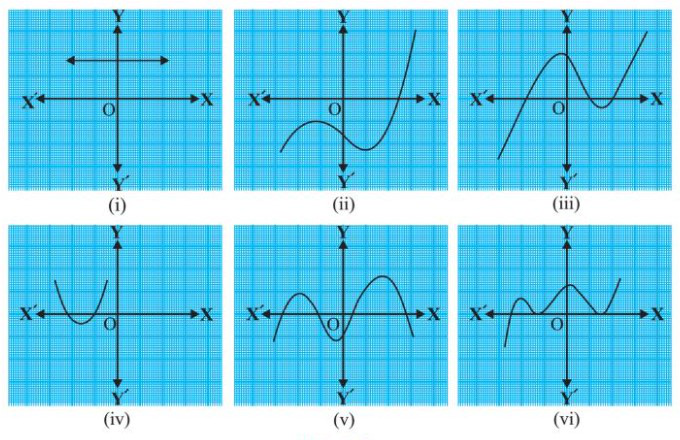GFG App
Open AppBrowser
Continue

# Class 10 NCERT Solutions- Chapter 2 Polynomials – Exercise 2.1

### Question 1. The graphs of y = p(x) are given in Fig. 2.10 below, for some polynomials p(x). Find the number of zeroes of p(x), in each case.Solution:

So, we have equation y = p(x). When p(x) = 0, y = 0.

That means we only need to find the number of times we get y = 0 in the graph.

In other words, the number of times the graph intersects the x-axis is the answer.

(i) 0. Because the graph doesn’t intersect the x-axis.

(ii) 1. Because the graph intersects the x-axis only at one point.

(iii) 3. Because the graph intersects the x-axis at three points.

(iv) 2. Because the graph intersects the x-axis at two points.

(v) 4. Because the graph intersects the x-axis at four points.

(vi) 3. Because the graph intersects the x-axis at three points.

My Personal Notes arrow_drop_up